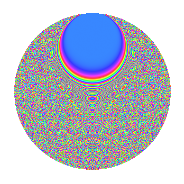# Properties

 Label 804.2.bdLevel 804 Weight 2 Character orbit bd Rep. character $$\chi_{804}(23,\cdot)$$ Character field $$\Q(\zeta_{66})$$ Dimension 2640 Newforms 1 Sturm bound 272 Trace bound 0

# Related objects

## Defining parameters

 Level: $$N$$ = $$804 = 2^{2} \cdot 3 \cdot 67$$ Weight: $$k$$ = $$2$$ Character orbit: $$[\chi]$$ = 804.bd (of order $$66$$ and degree $$20$$) Character conductor: $$\operatorname{cond}(\chi)$$ = $$804$$ Character field: $$\Q(\zeta_{66})$$ Newforms: $$1$$ Sturm bound: $$272$$ Trace bound: $$0$$

## Dimensions

The following table gives the dimensions of various subspaces of $$M_{2}(804, [\chi])$$.

Total New Old
Modular forms 2800 2800 0
Cusp forms 2640 2640 0
Eisenstein series 160 160 0

## Trace form

 $$2640q - 42q^{4} - 19q^{6} - 32q^{9} + O(q^{10})$$ $$2640q - 42q^{4} - 19q^{6} - 32q^{9} - 46q^{10} - 24q^{12} - 88q^{13} - 26q^{16} - 23q^{18} - 128q^{22} - 22q^{24} + 160q^{25} + 44q^{28} - 18q^{30} - 44q^{33} - 6q^{34} - 35q^{36} - 48q^{37} - 92q^{40} - 10q^{42} + 40q^{45} + 46q^{46} - 11q^{48} - 216q^{49} - 76q^{52} + 129q^{54} - 42q^{57} - 56q^{58} - 90q^{60} - 80q^{61} - 48q^{64} - 74q^{66} - 4q^{69} + 96q^{70} - 20q^{72} - 216q^{73} + 76q^{76} - 66q^{78} - 16q^{81} + 20q^{82} - 55q^{84} - 104q^{85} - 30q^{88} - 64q^{90} - 192q^{93} - 32q^{94} - 205q^{96} - 64q^{97} + O(q^{100})$$

## Decomposition of $$S_{2}^{\mathrm{new}}(804, [\chi])$$ into irreducible Hecke orbits

Label Dim. $$A$$ Field CM Traces $q$-expansion
$$a_2$$ $$a_3$$ $$a_5$$ $$a_7$$
804.2.bd.a $$2640$$ $$6.420$$ None $$0$$ $$0$$ $$0$$ $$0$$